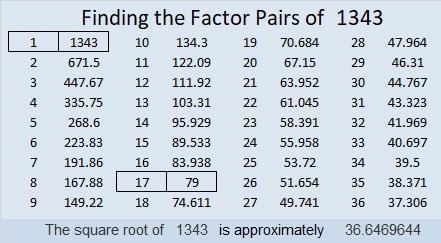# 1343 Level TWO Puzzles OnlyLevel 2 puzzles are much more interesting-looking than level 1 puzzles, but they are still relatively easy for beginners to solve. I decided to put all the level 2 puzzles from 2018 into one collection. You can use the image I put at the top of the post to work on solving them or you can find the complete collection at Level 2’s from 2018.

Now I’ll write a little bit about the number 1343:

• 1343 is a composite number.
• Prime factorization: 1343 = 17 × 79
• 1343 has no exponents greater than 1 in its prime factorization, so √1343 cannot be simplified.
• The exponents in the prime factorization are 1, and 1. Adding one to each exponent and multiplying we get (1 + 1)(1 + 1) = 2 × 2 = 4. Therefore 1343 has exactly 4 factors.
• The factors of 1343 are outlined with their factor pairs in the graphic below.1343 is the hypotenuse of a Pythagorean triple:
632-1185-1343 which is (8-15-17) times 79

OEIS.org informs us that 1343 is 16 numbers away from the closest prime number, and it is the smallest number that can make that claim.

This site uses Akismet to reduce spam. Learn how your comment data is processed.